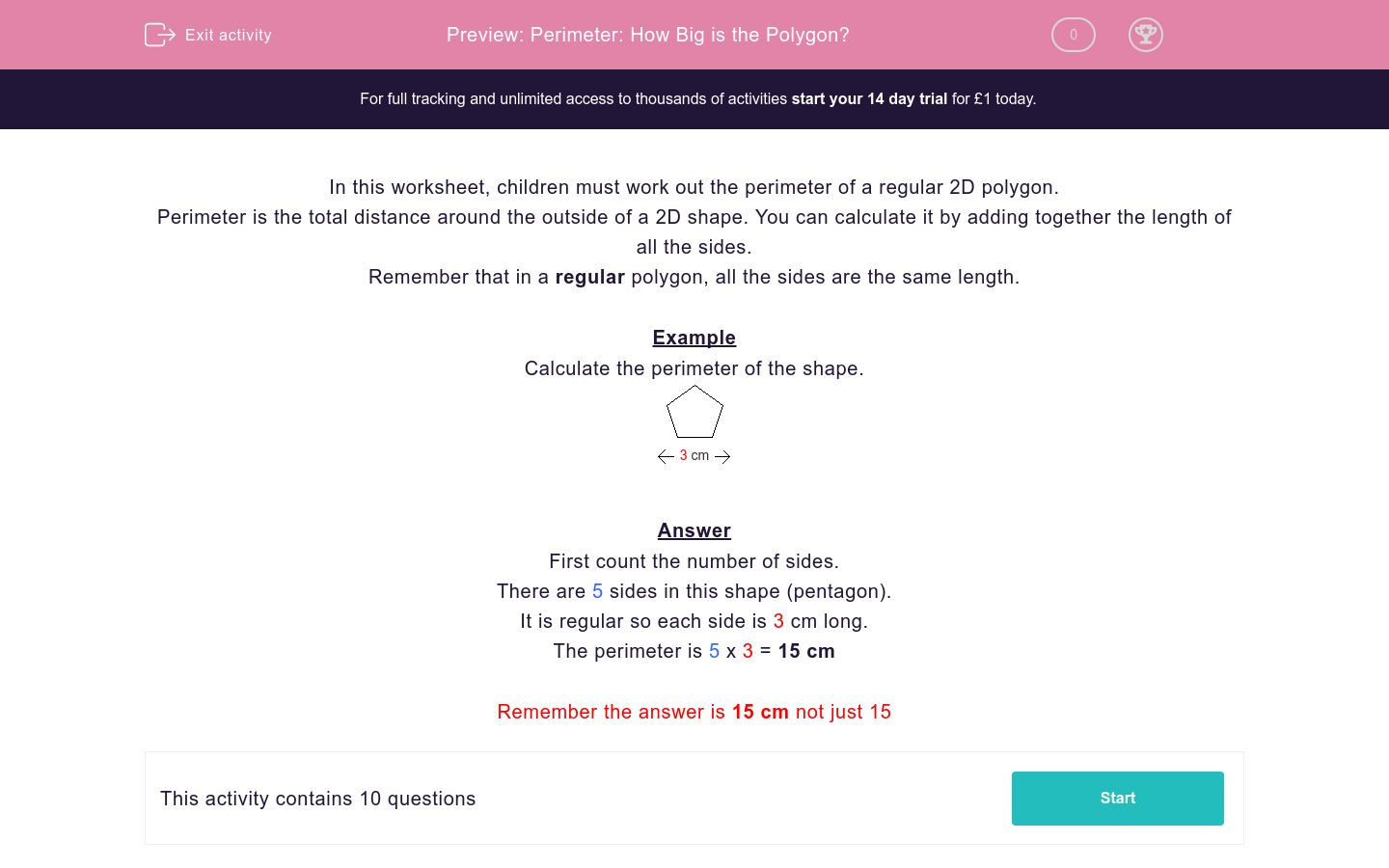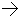# Perimeter: How Big is the Polygon?

In this worksheet, students work out the perimeter of a regular 2D polygon.Key stage:  KS 2

Curriculum topic:   Maths and Numerical Reasoning

Curriculum subtopic:   2D Shapes: Triangles, Quadrilaterals and Polygons

Difficulty level:### QUESTION 1 of 10

In this worksheet, children must work out the perimeter of a regular 2D polygon.

Perimeter is the total distance around the outside of a 2D shape. You can calculate it by adding together the length of all the sides.

Remember that in a regular polygon, all the sides are the same length.

Example

Calculate the perimeter of the shape.3 cmFirst count the number of sides.

There are 5 sides in this shape (pentagon).

It is regular so each side is 3 cm long.

The perimeter is 5 x 3 = 15 cm

Remember the answer is 15 cm not just 15

Calculate the perimeter of the shape.10 cmCalculate the perimeter of the shape.5 cmCalculate the perimeter of the shape.6 cmCalculate the perimeter of the shape.10 cmCalculate the perimeter of the shape.10 cmCalculate the perimeter of the shape.8 cmCalculate the perimeter of the shape.6 cmCalculate the perimeter of the shape.5 cmCalculate the perimeter of the shape.9 cmCalculate the perimeter of the shape.7 cm• Question 1

Calculate the perimeter of the shape.10 cm70cm
70 cm
• Question 2

Calculate the perimeter of the shape.5 cm25 cm
25cm
• Question 3

Calculate the perimeter of the shape.6 cm48cm
48 cm
• Question 4

Calculate the perimeter of the shape.10 cm80 cm
80cm
• Question 5

Calculate the perimeter of the shape.10 cm100cm
100 cm
• Question 6

Calculate the perimeter of the shape.8 cm72 cm
72cm
• Question 7

Calculate the perimeter of the shape.6 cm48 cm
48cm
• Question 8

Calculate the perimeter of the shape.5 cm40cm
40 cm
• Question 9

Calculate the perimeter of the shape.9 cm81cm
81 cm
• Question 10

Calculate the perimeter of the shape.7 cm49cm
49 cm
---- OR ----

Sign up for a £1 trial so you can track and measure your child's progress on this activity.

### What is EdPlace?

We're your National Curriculum aligned online education content provider helping each child succeed in English, maths and science from year 1 to GCSE. With an EdPlace account you’ll be able to track and measure progress, helping each child achieve their best. We build confidence and attainment by personalising each child’s learning at a level that suits them.

Get started